Courses

# Auto Transformer Electrical Engineering (EE) Notes | EduRev

## Electrical Engineering (EE) : Auto Transformer Electrical Engineering (EE) Notes | EduRev

The document Auto Transformer Electrical Engineering (EE) Notes | EduRev is a part of the Electrical Engineering (EE) Course Basic Electrical Technology.
All you need of Electrical Engineering (EE) at this link: Electrical Engineering (EE)

Goals of the lesson

In this lesson we shall learn about the working principle of another type of transformer called autotransformer and its uses. The differences between a 2-winding and an autotransformer will be brought out with their relative advantages and disadvantages. At the end of the lesson some objective type questions and problems for solving are given.

Key Words: tapping’s, conducted VA, transformed VA.

After going through this section students will be able to understand the following.

1. Constructional differences between a 2-winding transformer and an autotransformer.
2. Economic advantages/disadvantages between the two types.
3. Relative advantages/disadvantages of the two, based on technical considerations.
4. Points to be considered in order to decide whether to select a 2-winding transformer or an autotransformer.
5. The difference between an autotransformer and variac (or dimmerstat).
6. The use of a 2-winding transformer as an autotransformer.
7. The connection of three identical single phase transformers to be used in 3-phase system.

Introduction

So far we have considered a 2-winding transformer as a means for changing the level of a given voltage to a desired voltage level. It may be recalled that a 2-winding transformer has two separate magnetically coupled coils with no electrical connection between them. In this lesson we shall show that change of level of voltage can also be done quite effectively by using a single coil only. The idea is rather simple to understand. Suppose you have a single coil of 200 turns (= NBC) wound over a iron core as shown in figure 27.1. If we apply an a.c voltage of 400 V, 50 Hz to the coil (between points B and C), voltage per turn will be 400/200 = 2 V. If we take out a wire from one end of the coil say C and take out another wire tapped from any arbitrary point E, we would expect some voltage available between points E and C. The magnitude of the voltage will obviously be 2 × NEC where NEC is the number of turns between points E and C. If tapping has been taken in such a way that NEC = 100, voltage between E and C would be 200 V. Thus we have been able to change 400 V input voltage to a 200 V output voltage by using a single coil only. Such transformers having a single coil with suitable tapings are called autotransformers.   It is possible to connect a conventional 2-winding transformer as an autotransformer or one can develop an autotransformer as a single unit.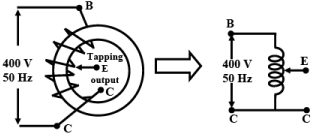Figure 27.1: Transformer with a single coil

2-winding transformer as Autotransformer

Suppose we have a single phase 200V/100V, 50Hz, 10kVA two winding transformer with polarity markings. Then the coils can be connected in various ways to have voltage ratios other than 2 also, as shown in figure 27.2.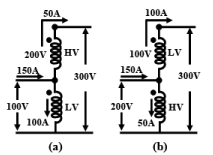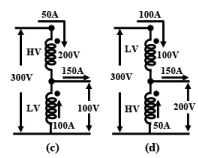Figure 27.2:  A two winding transformer connected as an autotransformer   in variou s ways.

Let us explain the one of the connections in figure 27.2(a) in detail. Here the LV and the HV sides are connected in additive series. For rated applied voltage (100V) across the LV winding, 200V must be induced across the HV winding. So across the whole combination we shall get a voltage of 300V. Thus the input voltage is stepped up by a factor of 3 (300 V/100 V). Now how much current can be supplied to a load at 300 V?  From the given rating of the transformer we know, IHV rated = 50 A and ILV rated = 100 A.  Therefore for safe operation of the transformer, these rated currents should not be exceeded in HV and LV coils.  Since the load is in series with the HV coil, 50A current can be safely supplied. But a current of 50A in the HV demands that the LV winding current must be 100A and in a direction as shown, in order to keep the flux in the core constant. Therefore by applying KCL at the junction, the current drawn from the supply will be 150A. Obviously the kVA handled by the transformer is 30 kVA and without overloading either of the windings. It may look a bit surprising because as a two winding transformer its rating is only 10 kVA. The explanation is not far to seek. Unlike a two winding transformer, the coils here are connected electrically. So the kVA transferred from supply to the load side takes place both inductively as well as conductively – 10kVA being transferred inductively and remaining 20kVA transferred conductively. The other connections shown in (b), (c) and (d) of figure 27.2 can similarly explained and left to the reader to verify.

Autotransformer as a single unit

Look at the figure 27.3 where the constructional features of an auto transformer is shown. The core is constructed by taking a rectangular long strip of magnetic material (say CRGO) and rolled to give the radial thickness. Over the core, a continuous single coil is wound the free terminals of which are marked as C and A. A carbon brush attached to a manually rotating handle makes contact with different number of turns and brought out as a terminal, marked E. The number of turns between E & C, denoted by NEC can be varied from zero to a maximum of total number of turns between A & C i.e, NAC. The output voltage can be varied smoothly from zero to the value of the input voltage simply by rotating the handle in the clockwise direction.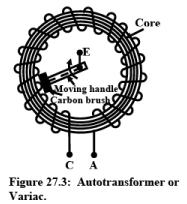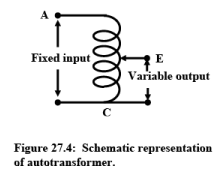This type of autotransformers are commercially known as varic or dimmerstat and is an important piece of equipment in any laboratory.  Now we find that to change a given voltage V1 to another level of voltage V2 and to transfer a given KVA from one side to the other, we have two choices namely by using a Two Winding Transformer or by using an Autotransformer. There are some advantages and disadvantages associated with either of them. To understand this aspect let us compare the two types of transformers in equal terms. Let,

Input voltage  = V1

Output voltage required across the load  = V2

Rated current to be supplied to the load  = I2

Current drawn from the supply at rated condition  = I1

KVA to be handled by both types of transformers  = V1I1 = V2I2

The above situation is pictorially shown in figures 27.5(a) and (b). Let for the two winding transformer,

For the two winding transformer:

Primary number of turns  = N1

Secondary number of turns  = N2

For the autotransformer:

Number of turns between A & C = N1

Number of turns between E & C  = N2

Therefore, number of turns between A & E  = N1 – N2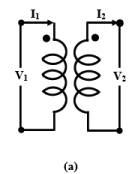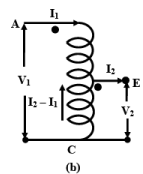Figure 27.5:  A two winding transformer and an autotransformer of same rating.

Let us now right down the mmf balance equation of the transformers.

For the two winding transformer:

MMF balance equation is N1I1  = N2I2

For the autotransformer:

MMF balance equation is (N1 – N2)I1 = N2(I2 – I1)

or, N1I1  = N2I2

It may be noted that in case of an autotransformer, the portion EC is common between the primary and the secondary. At loaded condition current flowing through NEC is (I2 – I1). Therefore, compared to a two winding transformer lesser cross sectional area of the conductor in the portion EC can be chosen, thereby saving copper. We can in fact find out the ratio of amount of copper required in two types of transformers noting that the volume of copper required will be proportional to the product of current and the number of turns of a particular coil.  This is because, length of copper wire is proportional to the number of turns and crossectional area of wire is proportional to the current value i.e.,

Volume of copper ∝ length of the wire × cross sectional area of copper wire

∝ N × I

Hence,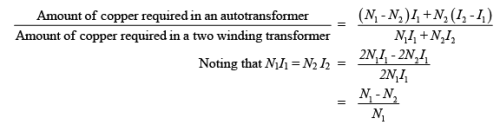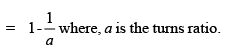Here we have assumed that N1 is greater than N2 i.e., a is greater than 1. The savings will of course be appreciable if the value of a is close to unity. For example if a = 1.2, copper required for autotransformer will be only 17% compared to a two winding transformer, i.e, saving will be about 83%. On the other hand, if a = 2, savings will be only 50%. Therefore, it is always economical to employ autotransformer where the voltage ratio change is close to unity. In fact autotransformers could be used with advantage, to connect two power systems of voltages say 11 kV and 15 kV.

Three similar single units of autotransformers could connected as shown in the figure 27.6 to get variable balanced three phase output voltage from a fixed three phase voltage. Such connections are often used in the laboratory to start 3-phase induction motor at reduced voltage.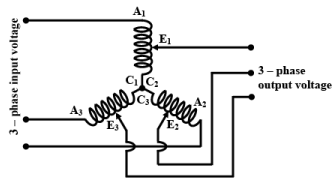Figure 27.6:  3 – phase autotransformer connection

Apart from being economical, autotransformer has less leakage flux hence improved regulation. Copper loss in the common portion of the winding will be less, so efficiency will be slightly more. However its one major disadvantage is that it can not provide isolation between HV and LV side. In fact, due to an open circuit in the common portion between E & C, the voltage on the load side may soot up to dangerously high voltage causing damage to equipment. This unexpected rise in the voltage on the LV side is potentially dangerous to the personnel working on the LV side.

Offer running on EduRev: Apply code STAYHOME200 to get INR 200 off on our premium plan EduRev Infinity!

62 docs|59 tests

,

,

,

,

,

,

,

,

,

,

,

,

,

,

,

,

,

,

,

,

,

;